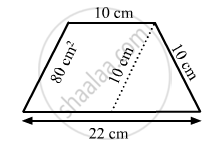Share

# In Fig. 20.38, a Parallelogram is Drawn in a Trapezium, the Area of the Parallelogram is 80 Cm2, Find the Area of the Trapezium. - Mathematics

Course
ConceptArea of Trapezium

#### Question

In Fig. 20.38, a parallelogram is drawn in a trapezium, the area of the parallelogram is 80 cm2, find the area of the trapezium.#### Solution

The given figure is:From above figure, it is clear that the length of the parallel sides of the trapezium are 22 cm and 10 cm.

Also, it is given that the area of the parallelogram is 80 cm2 and its base is 10 cm.

We know:

Area of parallelogram=Base x Height

∴ 80 = 10 x Height

$\text{ Height }=\frac{80}{10}= 8 cm$

So, now we have the distance between the parallel sides of trapezium, which is equal to 8 cm.

$\therefore\text{ Area of trapezium }=\frac{1}{2}\times(\text{ Sum of the parallel sides })\times(\text{ Distance between the parallel sides })$

$= \frac{1}{2}\times(22+10)\times(8)$

${=128 cm}^2$

Is there an error in this question or solution?

#### APPEARS IN

RD Sharma Solution for Mathematics for Class 8 by R D Sharma (2019-2020 Session) (2017 to Current)
Chapter 20: Mensuration - I (Area of a Trapezium and a Polygon)
Ex. 20.2 | Q: 19 | Page no. 24

#### Video TutorialsVIEW ALL 

Solution In Fig. 20.38, a Parallelogram is Drawn in a Trapezium, the Area of the Parallelogram is 80 Cm2, Find the Area of the Trapezium. Concept: Area of Trapezium.
S